# How to Find Area of a Parallelogram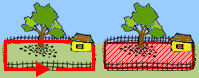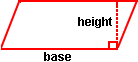The area of a polygon is the number of square units inside the polygon. Area is 2-dimensional like a carpet or an area rug.

A parallelogram is a 4-sided shape formed by two pairs of parallel lines. Opposite sides are equal in length and opposite angles are equal in measure. To find the area, multiply the base by the height. The formula is:

A = B * H where B is the base, H is the height, and * means multiply.

The base and height of a parallelogram must be perpendicular. However, the lateral sides of a parallelogram are not perpendicular to the base. Thus, a dotted line is drawn to represent the height. Let's look at some examples involving the area of a parallelogram.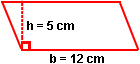Example 1: Find the area of this parallelogram with a base of 12 centimeters and a height of 5 centimeters.

Solution:

A = B * H

A = (12 cm) · (5 cm)

A = 60 cm2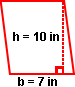Example 2: Find the area of this parallelogram with a base of 7 inches and a height of 10 inches.

Solution:

A = B * H

A = (7 in) · (10 in)

A = 70 in2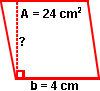Example 3: The area of a parallelogram is 24 square centimeters and the base is 4 centimeters. Find the height.

Solution:

A = B * H

24 cm2 = (4 cm) · H

24 cm2 ÷ (4 cm) = H

H = 6 cmSummary: Given the base and height of a parallelogram, we can find the area. Given the area and either the base or the height, we can find the missing dimension. The formula for area of a parallelogram is:

A = B * H where B is the base, H is the height, and * means multiply.

### Exercises

Directions: Read each question below. Click once in an ANSWER BOX and type in your answer; then click ENTER. Your answers should be given as whole numbers greater than zero. After you click ENTER, a message will appear in the RESULTS BOX to indicate whether your answer is correct or incorrect. To start over, click CLEAR.

 1 Find the area of a parallelogram with a base of 8 feet and a height of 3 feet.  ANSWER BOX:  A =  ft2  RESULTS BOX:
 2 Find the area of a parallelogram with a base of 4 meters and a height of 9 meters.  ANSWER BOX:  A =  m2  RESULTS BOX:
 3 The area of a parallelogram is 64 square inches and the height is 16 inches. Find the base.  ANSWER BOX:  B =  in  RESULTS BOX:
 4 A parallelogram has an area of 54 square centimeters and a base of 6 centimeters. Find the height.  ANSWER BOX:  H =  cm  RESULTS BOX:
 5 A parallelogram-shaped garden has an area of 42 square yards and a height of 6 yards. Find the base.  ANSWER BOX:  B =  yd  RESULTS BOX: